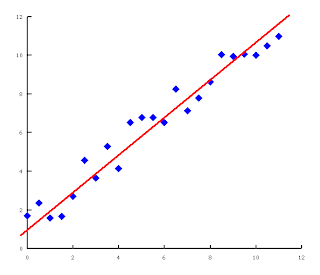## 2010-08-04

### Manifold Learning - part 2

Dimensionality Reduction

Trying to make sense of 19,200 dimensions is asking for trouble. Fortunately for us poor humans, most data are constrained in some way. For example, the data varies but it doesn't vary along all 19,200 dimensions at the same time; it varies along some of the dimensions, some of the time. If we know how and when the data changes, we can approximate our data with a smaller set of dimensions. This is the known as dimensionality reduction.

An Illustrated Example

Let's take a two-dimensional example. Let's say the data we have collected come in pairs, and when we plot them it looks like this:Unfortunately, the analytical tools that we have only work in one-dimension. We need to reduce the number of dimensions before we can analyse it. Fortunately for us, it seems the data we have (almost) fall along a straight line.Let's rotate our plot such that the line becomes the new X-axis. It's still the same data, we just changed the way we look at it. Notice that the data (blue squares) are very close to the new axis (red line).If the variation along the new Y-axis is much, much smaller than the variation along the new X-axis, we can approximate our data by it's projection along the new X-axis. We can pretend that the projections (red circles) are our data (blue squares) if our data is very, very close to the new X-axis (red line)*.We can now use the projections in our tools because it has only one dimension. We have reduced the number of dimensions of our data from two to one. Yes, errors will be introduced since the projections are not the same as our data. As long as the variations along one (new) axis is much, much larger than the other (new) axis, the error will be small.Principal Component Analysis (PCA) is one such method that does this, applicable in many problem domains.

* Let's ignore what we mean by "very, very close" for now.